ClockWork Games
We have moved to http://clockworkgames.ipbfree.com Go there Register & Post!..

# [ORGANIZED]Hez Values

#### 1shotkillRetired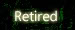volcom89 wrote:omg thank you so much..i needed the hex value numbers for a delete code on nfs carbon.now i can delete the hackers who deleted my acc

np

#### volcom89omg thank you so much..i needed the hex value numbers for a delete code on nfs carbon.now i can delete the hackers who deleted my acc

#### 1shotkillRetiredIvIr.IvIedicMan wrote:Hell yea nice job REP+
kk ty#### IvIr.IvIedicMan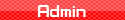Hell yea nice job REP+

_________________
[align=center]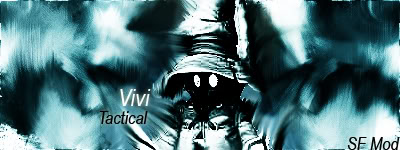[/align][align=center]Goto my new website at:ClockWorkGames[/align]

And dont forget to Rep me if I ever help!

#### 1shotkillRetired#Imposter (USA)
0x00492478 0x00000000
0x0049247C 0x00000000
0x00492480 0x00000000
0x00492484 0x00000000
0x00492488 0x00000000
#Meme
;DMA CODE 160 FTB2
0xFFFFFFFF 0x0055BD88
0x00000000 0x00000000
0x00000004 0x00000000
0x00000008 0x00000000
0x0000000c 0x00000000
0x00000010 0x00000000
0x00000014 0x00000000
0x00000018 0x00000000
0x0000001C 0x00000000
0x00000020 0x00000000
0x00000024 0x00000000
0x00000028 0x00000000
0x0000002c 0x00000000
#Clan MEME
;DMA CODE 160 FTB2
0xFFFFFFFF 0x0055BD88
0x00000000 0x00000000
0x00000004 0x00000000
0x00000008 0x00000000
#OSD Top Room
0x00513D78 0x00000000
0x00513D7C 0x00000000
0x00513D80 0x00000000
0x00513D84 0x00000000
0x00513D88 0x00000000
0x00513D8C 0x00000000
0x00513D90 0x00000000
0x00513D94 0x00000000
0x00513D98 0x00000000
0x00513D9C 0x00000000
0x00513DA0 0x00000000
0x00513DA4 0x00000000
0x00513DA8 0x00000000
0x00513DAC 0x00000000
0x00513DB0 0x00000000
0x00513DB4 0x00000000

Here you go the Hex values that i organized!!
Code:

Cap Letters: Lower Letters: Numbers:
A=41 a=61 0=30
B=42 b=62 1=31
C=43 c=63 2=32
D=44 d=64 3=33
E=45 e=65 4=34
F=46 f=66 5=35
G=47 g=67 6=36
H=48 h=68 7=37
I=49 i=69 8=38
J=4A j=6A 9=39
K=4B k=6B
L=4C l=6C
M=4D m=6D
N=4E n=6E
O=4F o=6F
P=50 p=70
Q=51 q=71
R=52 r=72
S=53 s=73
T=54 t=74
U=55 u=75
V=56 v=76
W=57 w=77
X=58 x=78
Y=59 y=79
Z=5A z=7A

Code:

Symbols: Cap Euro Letters: Lower Euro Letters:
[SPACE]=20 À=c0c3 à=a0c3
!=21 Á=c1c3 á=a1c3
"=22 Â=c2c3 â=a2c3
#=23 Ã=c3c3 ã=a3c3
\$=24 Ä=c4c3 ä=a4c3
%=25 Å=c5c3 å=a5c3
&=26 Æ=c6c3 æ=a6c3
'=27 Ç=c7c3 ç=a7c3
(=28 È=c8c3 è=a8c3
)=29 É=c9c3 é=a9c3
*=2A Ê=cac3 ê=aac3
+=2B Ë=cbc3 ë=abc3
,=2C Ì=ccc3 ì=acc3
.=2E Î=cec3 î=aec3
/=2F Ï=cfc3 ï=afc3
:=3A Ñ=d1c3 ñ=b1c3
;=3B Ò=d2c3 ò=b2c3
<=3C Ó=d3c3 ó=b3c3
==3D Ô=d4c3 ô=b4c3
>=3E Õ=d5c3 õ=b5c3
?=3F Ö=d6c3 ö=b6c3
@=40 Ø=d8c3 ø=b8c3
[=5B Ù=d9c3 ù=b9c3
\=5C Ú=dac3 ú=bac3
]=5D Û=dbc3 û=bbc3
^=5E Ü=dcc3 ü=bcc3
_=5F Ÿ=b8c5 ÿ=bfc3
`=60 ß=9fc3
{=7B
|=7C
}=7D
~=7E

Code:

Euro STuff Other: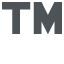=A284E2 #N=4E23
¡=A1 {*0}=7D302A7B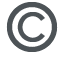=A9 {*1}=7D312A7B
«=AB {*2}=7D322A7B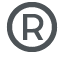=AE {tri}=7D6972747B
°=B0 {dUP}=7D5055647B
»=BB {dDown}=7D6E776F44647B
¿=BF {dRight}=7D7468676952647B
{dLeft}=7D7466654C647B
{Circ}=7D637269437B
{X}=7D587B
{sq}=7D71737B
{Select}=7D7463656C65537B
{Start}=7D747261747537B
{L1}=7D314C7B
{R1}=7D31527B
{Stick}=7D6B636974537B

Code:

Definitions:
{*0} = white
{*1} = red
{*2} = yellow
#N = next line
{tri} = Triangle
{Circ} = Circle
{X} = Cross
{sq} Square
{Select} = SELECT
{Start} = START
{L1} = Left Trigger
{R1} = Right Trigger
{Stick} = Analogue Stick